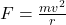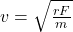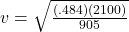## 5. A 905 kg test car travels around a 3.04 km circular track. If the magnitude of the centripetal force is 2100 N. What is the car’s speed?​

Question

5. A 905 kg test car travels around a 3.04 km circular track. If the magnitude of the centripetal force is 2100 N. What is the car’s speed?​

in progress 0
2 months 2021-07-30T17:08:39+00:00 1 Answers 5 views 0

Explanation:

The equation for centripetal force is. We have all the values we need except for the radius. We have the circumference of the circle, though, so we will solve for the radius using that and the fact that C = 2πr:

3.04 = 2(3.1415)r and

r = .484 m, to the correct number of sig fig’s.

Now that we have everything we need and isolating the v NOT squared:and filling in:. This answer will need 2 sig fig’s since 2100 has 2 sig fig’s in it. That means that the velocity of the test car is

1.1 m/sec